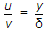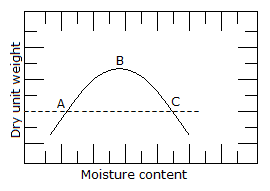# Civil Engineering - UPSC Civil Service Exam Questions

46.
In a laminar boundary layer, the velocity distribution can be assumed to be given, in usual notations, asWhich one of the following is the correct expression for the displacement thickness δ* for this boundary layer ?
δ* = δ
δ* = δ/2
δ* = δ/4
δ* = δ/6
Explanation:
No answer description is available. Let's discuss.

47.
In a plate load test, how is the ultimate load estimated from the load settlement curve on a log-log graph ?
Directly
By drawing tangents to the curve at the initial and final points
By the secant method
At 0.2 percent of the maximum settlement
Explanation:
No answer description is available. Let's discuss.

48.
The standard Proctor compaction curve of a clay is depicted in the above figure. Points A, B and C correspond to three compaction states of the soil, which fall on this curve. For which point(s) is the coefficient of permeability minimum ?A and C
A
B
C
Explanation:
No answer description is available. Let's discuss.

49.
In which one of the following grades of a highway is an emergency escape ramp provided ?
1 in 200